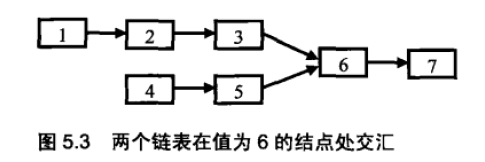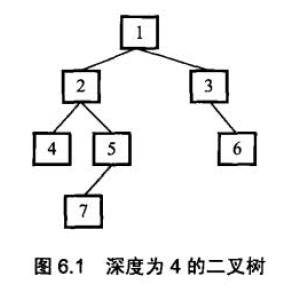# 算法题目（四）

## 一些算法题的解法

Posted by Ted on March 20, 2015

31、丑数

32、第一个只出现过一次的字符

33、数组中的逆序对

34、两个链表的第一个公共结点

35、数字在排序数组中出现的次数

36、二叉树的深度

37、数组中只出现一次的数字

38、和为S的两个数字

39、翻转单词顺序

40、左旋字符串

#### 31、丑数

``````int Min(int number1, int number2, int number3)
{
int min = (number1 < number2) ? number1 : number2;
min = (min < number3) ? min : number3;
return min;
}
int findUglyNum2(int index)
{
int *pUglyNumbers = new int[index];
pUglyNumbers = 1;
int nextUglyIndex = 1;

int *pMultiply2 = pUglyNumbers;
int *pMultiply3 = pUglyNumbers;
int *pMultiply5 = pUglyNumbers;

while(nextUglyIndex < index)
{
int min = Min(*pMultiply2*2, *pMultiply3*3,*pMultiply5*5);
pUglyNumbers[nextUglyIndex] = min;
while(*pMultiply2*2 <= pUglyNumbers[nextUglyIndex])
++pMultiply2;
while(*pMultiply3*3 <= pUglyNumbers[nextUglyIndex])
++pMultiply3;
while(*pMultiply5*5 <= pUglyNumbers[nextUglyIndex])
++pMultiply5;
++nextUglyIndex;
}
int ugly = pUglyNumbers[nextUglyIndex - 1];
delete[] pUglyNumbers;
return ugly;
}

int main()
{
time_t   begin2,end2;
begin2=clock();
cout<<findUglyNum2(1500)<<endl;
end2=clock();
cout<<"runtime:   "<<double(end2-begin2)/CLOCKS_PER_SEC<<endl;

return 0;
}
``````

#### 32、第一个只出现过一次的字符

``````#include "stdafx.h"
#include <iostream>
using namespace std;

/*

*/

char FindFirstChar(char* pString)
{
if(pString == NULL)
return '\0';

const int N = 256;
int b[N];
for(int i = 0; i < N; ++i)
{
b[i] = 0;
}

char* p = pString;
while (*p != '\0')
{
b[*(p++)]++;
}

p = pString;
while (*p != '\0')
{
if (b[*p] == 1)
{
return *p;
}
++p;
}
return '\0';

}
int main()
{
char* pString = "abcddeeff";
char c = FindFirstChar(pString);
cout<<c<<endl;

getchar();
return 0;
}
``````

#### 33、数组中的逆序对

1）总体的意思就是将数组分成两段，首先求段内的逆序对数量，比如下面两段代码就是求左右两端数组段内的逆序对数量

``````inversions+=InversePairsCore(arry,start,mid,temp);//找左半段的逆序对数目
inversions+=InversePairsCore(arry,mid+1,end,temp);//找右半段的逆序对数目
``````

（2）然后求段间的逆序对数量，如下面的代码

``````inversions+=MergeArray(arry,start,mid,end,temp);//在找完左右半段逆序对以后两段数组有序，然后找两段之间的逆序对。最小的逆序段只有一个元素。
``````

（3）然后在求段间逆序对的时候，我们分为arry[start…mid]和arry[mid+1…end]，然后设置两个指针ij分别指向两段数组的末尾元素，也就是i=mid，j=end。然后比较arry[i]和arry[j]，

1. 如果arry[i]>arry[j]，因为两段数组都是有序的，所以arry[i]>arry[mid+1…j]，这些都是逆序对，我们统计出的逆序对为j-(mid+1)+1=j-mid。并且将大数arry[i]放入临时数组temp[]当中，i往前移动
2. 如果arry[i]<arry[j]，则将大数arry[j]放入temp[]中，j往前移。
``````#include<iostream>
#include<stdlib.h>
using namespace std;

void printArray(int arry[],int len)
{
for(int i=0;i<len;i++)
cout<<arry[i]<<" ";
cout<<endl;
}
int MergeArray(int arry[],int start,int mid,int end,int temp[])//数组的归并操作
{
//int leftLen=mid-start+1;//arry[start...mid]左半段长度
//int rightLlen=end-mid;//arry[mid+1...end]右半段长度

int i=mid;
int j=end;
int k=0;//临时数组末尾坐标
int count=0;
//设定两个指针ij分别指向两段有序数组的头元素，将小的那一个放入到临时数组中去。
while(i>=start&&j>mid)
{
if(arry[i]>arry[j])
{
temp[k++]=arry[i--];//从临时数组的最后一个位置开始排序
count+=j-mid;//因为arry[mid+1...j...end]是有序的，如果arry[i]>arry[j]，那么也大于arry[j]之前的元素，从a[mid+1...j]一共有j-(mid+1)+1=j-mid

}
else
{
temp[k++]=arry[j--];
}
}
cout<<"调用MergeArray时的count："<<count<<endl;
while(i>=start)//表示前半段数组中还有元素未放入临时数组
{
temp[k++]=arry[i--];
}

while(j>mid)
{
temp[k++]=arry[j--];
}

//将临时数组中的元素写回到原数组当中去。
for(i=0;i<k;i++)
arry[end-i]=temp[i];

printArray(arry,8);//输出进过一次归并以后的数组，用于理解整体过程
return count;

}

int InversePairsCore(int arry[],int start,int end,int temp[])
{
int inversions = 0;
if(start<end)
{
int mid=(start+end)/2;
inversions+=InversePairsCore(arry,start,mid,temp);//找左半段的逆序对数目
inversions+=InversePairsCore(arry,mid+1,end,temp);//找右半段的逆序对数目
inversions+=MergeArray(arry,start,mid,end,temp);//在找完左右半段逆序对以后两段数组有序，然后找两段之间的逆序对。最小的逆序段只有一个元素。
}
return inversions;
}

int InversePairs(int arry[],int len)
{
int *temp=new int[len];
int count=InversePairsCore(arry,0,len-1,temp);
delete[] temp;
return count;
}

void main()
{
//int arry[]={7,5,6,4};
int arry[]={1,3,7,8,2,4,6,5};
int len=sizeof(arry)/sizeof(int);
//printArray(arry,len);
int count=InversePairs(arry,len);
//printArray(arry,len);
//cout<<count<<endl;
system("pause");
}
``````

#### 34、两个链表的第一个公共结点``````/*

*/
{
int len = 0;
while(pCur != NULL)
{
len++;
pCur = pCur->next;
}
return len;
}

/*

*/
{
return NULL;

int distance = len1-len2;
int shortLength = len2;
if(len1 < len2)
{
distance = len2-len1;
shortLength = len1;
}

int i;
for(i=0;i<distance;i++)
pListLong = pListLong->next;
for(i=0;i<shortLength;i++)
{
if(pListLong->data == pListShort->data)
break;
pListLong = pListLong->next;
pListShort = pListShort->next;
}
return pListLong;
}
``````

#### 35、数字在排序数组中出现的次数

``````#include<iostream>
using namespace std;

int searchFirstEqual(int a[],int n,int key)
{
int low=0,high=n-1,mid;
while(low<=high)
{
mid=(low+high)>>1;
if(a[mid]>=key)
high=mid-1;
else
low=mid+1;
}
if(low<n && a[low]==key) return low;
else
return -1;
}

int searchLastEqual(int arr[],int n,int key)
{
int low=0,high=n-1,mid;
while(low<=high)
{
mid=low+(high-low)/2;
if(arr[mid]<=key)
{
low=mid+1;
}
else
{
high=mid-1;
}
}

if(high>=0 && arr[high]==key) return high;
else
return -1;
}

int main()
{
int a[]={1,3,3,3,5,6};
int n=sizeof(a)/sizeof(a);

int start=searchFirstEqual(a,n,3);
int end=searchLastEqual(a,n,3);

cout<<start<<endl;
cout<<end<<endl;
cout<<end-start+1<<endl;
}
``````

#### 36、二叉树的深度``````int TreeDepth(BinaryTreeNode* pRoot) {
if (pRoot == NULL)
return 0;
int nLeft = TreeDepth(pRoot->m_pLeft);
int nRight = TreeDepth(pRoot->m_pRight);
return (nLeft>nRight) ? (nLeft+1):(nRight+1);
}
``````

#### 37、数组中只出现一次的数字

``````void FindNumsAppearOnce(vector<int> data,int* num1,int *num2) {
if(data.size()<2)   return ;

int size=data.size();
int temp=0;
for(int i=0;i<size;i++)//对数组中每个数字做异或
temp=temp^data[i];
if(temp==0)    return ;

int index=0;
while((temp&1)==0)//在整数temp的二进制表示中找到最右边是1的位
{
temp=temp>>1;
++index;
}

//根据数组的倒数第index位是否为1，将数组划分为两个子数组，并分别对两个子数组进行求异或，得到num1			和num2
*num1=*num2=0;
for(int i=0;i<size;i++)
{
if(IsBit(data[i],index))
*num1^=data[i];
else
*num2^=data[i];
}
}

//判断在num的二进制表示中从右边起的index位是不是1
bool IsBit(int num,int index)
{
num=num>>index;
return (num&1);
}
``````

#### 38、和为S的两个数字

``````typedef vector<int> vi;
class Solution {
public:
vi FindNumbersWithSum(const vi& a,int sum) {
vi res;
int n = a.size();
int i = 0, j = n - 1;
while(i < j){
if(a[i] + a[j] == sum){
res.push_back(a[i]);
res.push_back(a[j]);
break;
}
while(i < j && a[i] + a[j] > sum) --j;
while(i < j && a[i] + a[j] < sum) ++i;
}
return res;
}
};
``````

#### 39、翻转单词顺序

``````#include <iostream>
#include <string>

using namespace std;

string ReverseSentence(string str)
{
string res = "", tmp = "";

for(unsigned int i = 0; i < str.size(); ++i)
{
// 遇到空格
if(str[i] == ' ')
{
res = " " + tmp + res;
cout << tmp << "\n" <<endl;
tmp = "";
}
else
{
tmp += str[i];
}
}

// 最后一个字符
if(tmp.size())
res = tmp + res;

return res;
}

int main(void)
{
string str = "student. a am I";
cout<<ReverseSentence(str)<<endl;

return 0;
}
``````

#### 40、左旋字符串

``````#include <stdio.h>

void reverse(char a[],int l,int r){
int i = l;
int j = r;
for (; i < j ; i++,j--) {
char temp = a[i];
a[i] = a[j];
a[j] = temp;
}
printf("==%s\n",a);
}

void left_rever(char a[],int leng,int n){
reverse(a,0,n-1);
reverse(a,n,leng-1);
reverse(a,0,leng-1);
printf("%s",a);
}

int main(void)
{
char a[] = "abcdefg";
left_rever(a,7,2);
return 0;
}
``````

####